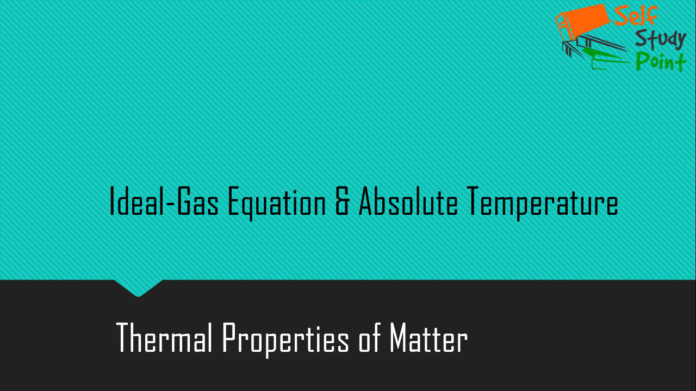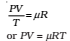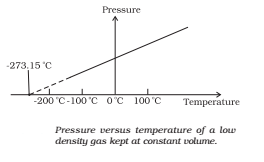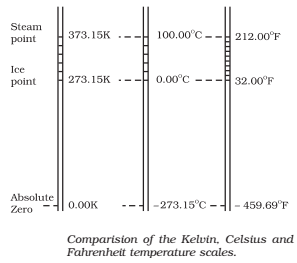## Ideal-Gas Equation

The ideal gas equation connecting pressure (P), volume (V) and absolute temperature (T) is PV = μ R T

since PV = constant and V/T = constant for a given quantity of gas, then PV/T should also be a constant. This relationship is known as ideal gas law or ideal-gas equation.where μ is the number of moles and R is the universal gas constant.

R = 8.31 J mol-1 K-1## Absolute Temperature

In the absolute temperature scale, the zero of the scale is the absolute zero of temperature – the temperature where every substance in nature has the least possible molecular activity. The Kelvin absolute temperature scale (T) has the same unit size as the Celsius scale (TC), but differs in the origin:

TC = T – 273.15The size of the unit for Kelvin temperature is the same Celsius degree, so temperature on these scales are related by

T = TC + 273.15